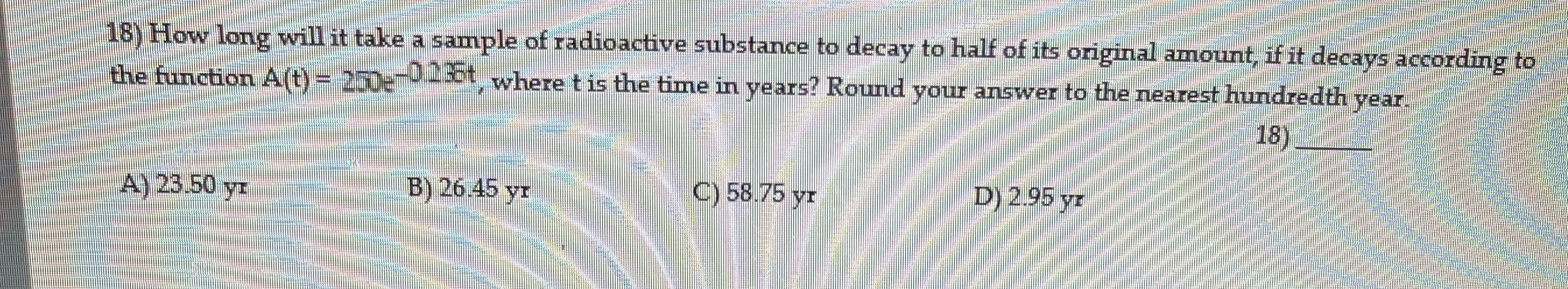### ¿Todavía tienes preguntas de matemáticas?

Pregunte a nuestros tutores expertos
Algebra
Pregunta18) How long will it take a sample of radioactive substance to decay to half of its original amount, if it decays according to the function $$A ( t ) = 250 e ^ { - D .236 t }$$ , where $$t$$ is the time in years? Round your answer to the nearest hundredth year.

A) $$23.50 y r$$  B) $$26.45 y r$$  C) $$58.75 y r$$ D) $$2.95 y r$$